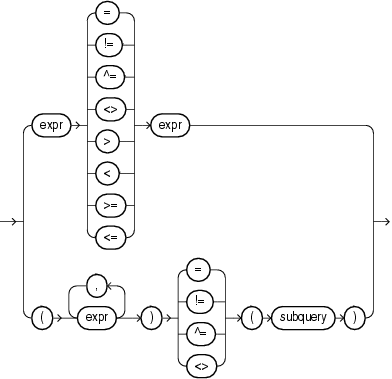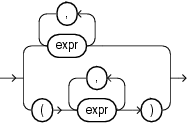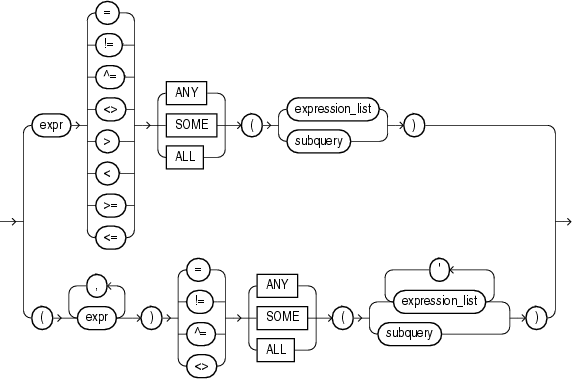# Comparison Conditions

Comparison conditions compare one expression with another. The result of such a comparison can be `TRUE`, `FALSE`, or `NULL`.

Large objects (LOBs) are not supported in comparison conditions. However, you can use PL/SQL programs for comparisons on `CLOB` data.

When comparing numeric expressions, Oracle uses numeric precedence to determine whether the condition compares `NUMBER`, `BINARY_FLOAT`, or `BINARY_DOUBLE` values. Refer to "Numeric Precedence" for information on numeric precedence.

Two objects of nonscalar type are comparable if they are of the same named type and there is a one-to-one correspondence between their elements. In addition, nested tables of user-defined object types, even if their elements are comparable, must have `MAP` methods defined on them to be used in equality or `IN` conditions.

Table 7-2 lists comparison conditions.

Table 7-2 Comparison Conditions

Type of Condition Purpose Example
```=
```

Equality test.

```SELECT *
FROM employees
WHERE salary = 2500
ORDER BY employee_id;
```
```!=
^=
< >
ÿ=
```

Inequality test. Some forms of the inequality condition may be unavailable on some platforms.

```SELECT *
FROM employees
WHERE salary != 2500
ORDER BY employee_id;
```
```>

<
```

Greater-than and less-than tests.

```SELECT * FROM employees
WHERE salary > 2500
ORDER BY employee_id;
SELECT * FROM employees
WHERE salary < 2500
ORDER BY employee_id;
```
```>=

<=
```

Greater-than-or-equal-to and less-than-or-equal-to tests.

```SELECT * FROM employees
WHERE salary >= 2500
ORDER BY employee_id;
SELECT * FROM employees
WHERE salary <= 2500
ORDER BY employee_id;
```
```ANY
SOME
```

Compares a value to each value in a list or returned by a query. Must be preceded by =, !=, >, <, <=, >=. Can be followed by any expression or subquery that returns one or more values.

Evaluates to `FALSE` if the query returns no rows.

```SELECT * FROM employees
WHERE salary = ANY
(SELECT salary
FROM employees
WHERE department_id = 30)
ORDER BY employee_id;
```
```ALL
```

Compares a value to every value in a list or returned by a query. Must be preceded by =, !=, >, <, <=, >=. Can be followed by any expression or subquery that returns one or more values.

Evaluates to `TRUE` if the query returns no rows.

```SELECT * FROM employees
WHERE salary >=
ALL ( 1400, 3000)
ORDER BY employee_id;
```

## Simple Comparison Conditions

A simple comparison condition specifies a comparison with expressions or subquery results.

simple_comparison_condition::=Description of the illustration simple_comparison_condition.gif

expression_list::=Description of the illustration expression_list.gif

If you use the lower form of this condition (with multiple expressions to the left of the operator), then you must use the lower form of the `expression_list`, and the values returned by the subquery must match in number and datatype the expressions in `expression_list`.

## Group Comparison Conditions

A group comparison condition specifies a comparison with any or all members in a list or subquery.

group_comparison_condition::=Description of the illustration group_comparison_condition.gif

expression_list::=Description of the illustration expression_list.gif

If you use the upper form of this condition (with a single expression to the left of the operator), then you must use the upper form of `expression_list`. If you use the lower form of this condition (with multiple expressions to the left of the operator), then you must use the lower form of `expression_list`, and the expressions in each `expression_list` must match in number and datatype the expressions to the left of the operator.